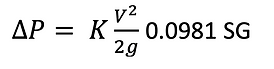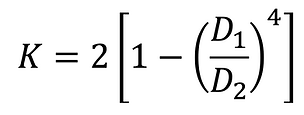## Pypley's Calculation Method

This page includes all the theory equations used in Pypley's calculation model.

We are continuously upgrading our software to increase its accuracy and to give useful experience for engineers, academics and technicians.

## 1.1 Darcy Equation

The Dacry equation for laminar flow is used at Reynolds number below 2100 where pipe friction factor varies with the reynolds number only :

If Re < 2100## 1.2 Colebrook Equation

The ColeBrook's equation is used with turbulent flow regions and it is solved in pypley by iteration at an accuracy of 0.000001.## 1.3 Pipe Pressure losses

After obtaining the pipe friction factor, pypley uses the equations below to calculate the pressure drop across the pipe length.metricimperial

## 2. Pipe Fitting losses

Pypley uses the same pressure drop equation above for fittings but the K values are obtained using the 3K and 2K method.

## 2.1 3K (Darby) method

The 3K method equation takes into account the variations in the Reynolds number and the geometry proportions in the calculated K value and that allows the K factor prediction to be more accurate in pressure drop calculations with viscous liquids and large pipe diameters.The table below includes all the fittings that Pypley calculates using the 3K method:

## 2.2 2K (Hooper) method

The 2K method equation takes into account the variations in Reynolds number in the calculated K value and that allows the K factor prediction to be more accurate in pressure drop calculations with viscous liquids with low Reynolds numbers.

The only fitting that uses the 2K method in Pypley is the Tilting disk check valve (K1 =1000, K∞ = 0.5)

Below is the 2K equation used in Pypley.## 2.3 Square Reduction

Pypley applies square reduction automatically whenever the next pipe is smaller than the previous one. The option of using tapered or rounded reductions will be included in the later iterations of the software.

Pypley uses the same pressure drop equations in section 1.3 for metric and imperial units. The K factor is calculated using the equations below that takes into account variations in the Reynolds number, the pipe friction factor and diameters :

If Re1 < 2500If Re1 > 2500## 2.4 Square Expansion

Just like the reduction Pypley applies square expansion automatically whenever the next pipe is bigger than the previous one. The option of using tapered or rounded expansions will be included in the later iterations of the software.

Pypley will also uses the same pressure drop equations in section 1.3 for metric and imperial units. The K factor is calculated using the equations below that takes into account the pipe friction factor and diameters:

If Re1 < 4000If Re1 > 4000Page Still Under Progress